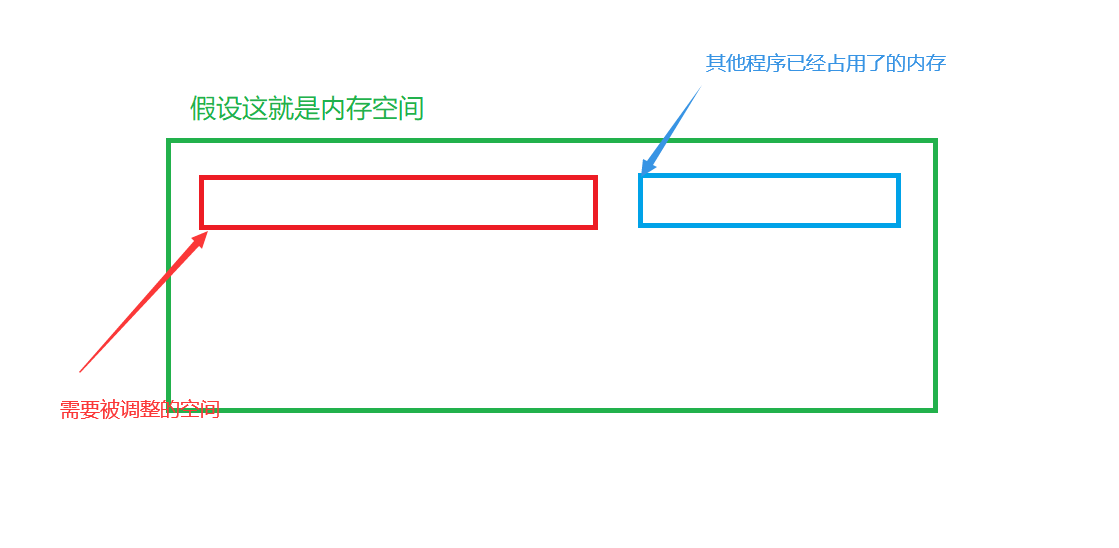C語言中關於動態內存分配的詳解

【C語言】動態內存分配

c語言中動態分配內存的函數，可能有些初學c語言的人不免要問瞭：我們為什麼要通過函數來實現動態分配內存呢？

1）棧：在棧裡面儲存一些我們定義的局部變量以及形參（形式參數）；

2）字符常量區：主要是儲存一些字符常量，比如：char *p=”hello world”;其中”hello world”就儲存在字符常量區裡面；

3）全局區：在全局區裡儲存一些全局變量和靜態變量；• 靜態內存是有系統自動分配，由系統自動釋放。 靜態內存是在棧分配的。（例如：函數裡的局部變量）
• 動態內存是由程序員手動分配，手動釋放。 動態內存是在堆分配的。（例如：用C語言寫鏈表時，需要自己對Node結點分配內存空間）

一、malloc 與free函數

void* **malloc( size_t ** size);

void free(void* memblock) ；

#include <stdlib.h>
#include <stdio.h>
int main()
{
//開辟10個int類型的空間
int* arr = (int*)malloc(10 * sizeof(int)); //切記這裡給的大小，是10  *  int（4個字節）
int i = 0;
if (arr == NULL)
{
perror("malloc"); //有可能，malloc開辟空間失敗，則malloc會返回NULL
return 1;
}

for (i = 0; i < 10; i++)
*(arr + i) = i; //放入數據 0 …… 9

for (i = 0; i < 10; i++)
printf("%d ",*(arr + i));

//記得釋放所開辟的空間
free(arr);
return 0;
}

二、calloc

void* calloc (size_t num， size_t** size );

​ size_ size, 每一個元素，所占用的內存空間是多少個字節。

#include <stdlib.h>
#include <stdio.h>

int main()
{
//還是申請10個int類型的內存空間
int* arr = (int*)calloc(10, sizeof(int));
if (arr == NULL)
{
perror("calloc"); //calloc開辟空間的話，會返回NULL
return 1;
}

//不做賦值運算，直接輸出剛開辟的空間，看是否是已經初始化為0瞭
int i = 0;
for (i = 0; i < 10; i++)
printf("%d ",*(arr + i));

//記得釋放空間
free(arr);
return 0;
}

三、realloc

void* **realloc(*void memblock， size_t size);

• memblock是需要調整的內存地址
• size調整之後新大小
• 返回值為調整之後的內存起始位置。
• 這個函數調整原內存空間大小的基礎_上,還會將原來內存中的數據移動到新的空間。
• realloc在調整內存空間的是存在兩種情況:

​ 情況1 :原有空間之後有足夠大的空間int main()
{
int* arr = (int*)malloc(5 * sizeof(int)); //先開辟5個int類型的空間
if (arr == NULL)
return 1;

//此時，我覺得malloc開辟的空間小瞭，我想增加
arr = (int*) realloc(arr, 10);

free(arr);
return 0;
}

int main()
{
int* arr = (int*)malloc(5 * sizeof(int)); //先開辟5個int類型的空間
if (arr == NULL)
return 1;

//此時，我覺得malloc開辟的空間小瞭，我想增加
int* tmp = NULL;
tmp = (int*) realloc(arr, 10);
if (tmp != NULL)
arr = tmp;

free(arr);
return 0;
}

四、常見的動態內存的錯誤

• 對NULL進行解引用操作
int main()
{
int* arr = (int*)malloc(10 * sizeof(int));
*arr = 10; //沒有對arr進行NULL的判斷
free(arr);
return 0;
}
• 對非動態內存分配的空間進行free釋放
int main()
{
int a = 10;
int* pa = &a;
free(pa); //pa指針，並不是malloc等函數開辟的空間，不能使用free釋放，系統會自動回收的
return 0;
}
• 使用free函數釋放一塊動態分配空間的一部分
int main()
{
int* arr = (int*)malloc(10 * sizeof(int));
if (arr == NULL)
return 1;
arr++; //此時，arr向後跳瞭4個字節
free(arr); //現在再去釋放空間，最前面的4個字節的空間就沒有釋放到，會報錯
return 0;
}
• 對同一塊內存空間進行多次釋放
int main()
{
int* arr = (int*)malloc(10 * sizeof(int));
if (arr == NULL)
return 1;

free(arr);
free(arr); //重復釋放瞭
return 0;
}
• 動態開辟的空間忘記釋放（內存泄漏）
int main()
{
int* arr = (int*)malloc(10 * sizeof(int));
if (arr == NULL)
return 1;

//沒有釋放空間，會造成內存泄漏
//造成內存泄漏，有很多原因，例如，在調用其他函數時，想傳回到本函數，指針沒用正確，導致開辟的空間沒有傳回來等等
return 0;
}

main()
{
int* arr = (int*)malloc(10 * sizeof(int));
if (arr == NULL)
return 1;

}

//沒有釋放空間，會造成內存泄漏
//造成內存泄漏，有很多原因，例如，在調用其他函數時，想傳回到本函數，指針沒用正確，導致開辟的空間沒有傳回來等等
return 0;